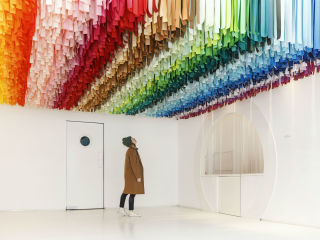# Color FactoryPhoto courtesy of Color Factory

Color Factory is a collaborative interactive exhibit that features participatory installations of colors, hues that invite curiosity, discovery, and play. A collection of artists, creatives, designers, and makers have teamed up to tell their unique color stories and engage all of the senses in unexpected ways.

### Event Details

#### When

12.16.19 | 9:30 am
12.17.19 | 9:30 am
12.19.19 | 9:30 am
+ see all dates and times
12.20.19 | 9:30 am
12.21.19 | 9:30 am
12.22.19 | 9:30 am
12.23.19 | 9:30 am
12.24.19 | 9:30 am
12.26.19 | 9:30 am
12.27.19 | 9:30 am
12.28.19 | 9:30 am
12.29.19 | 9:30 am
12.30.19 | 9:30 am
12.31.19 | 9:30 am
1.2.20 | 9:30 am
1.3.20 | 9:30 am
1.4.20 | 9:30 am
1.5.20 | 9:30 am
1.6.20 | 9:30 am
1.7.20 | 9:30 am
1.9.20 | 9:30 am
1.10.20 | 9:30 am
1.11.20 | 9:30 am
1.12.20 | 9:30 am
1.13.20 | 9:30 am
1.14.20 | 9:30 am
1.16.20 | 9:30 am
1.17.20 | 9:30 am
1.18.20 | 9:30 am
1.19.20 | 9:30 am
1.20.20 | 9:30 am
1.21.20 | 9:30 am
1.23.20 | 9:30 am
1.24.20 | 9:30 am
1.25.20 | 9:30 am
1.26.20 | 9:30 am
1.27.20 | 9:30 am
1.28.20 | 9:30 am
1.30.20 | 9:30 am
1.31.20 | 9:30 am
2.1.20 | 9:30 am
2.2.20 | 9:30 am
2.3.20 | 9:30 am
2.4.20 | 9:30 am
2.6.20 | 9:30 am
2.7.20 | 9:30 am
2.8.20 | 9:30 am
2.9.20 | 9:30 am
2.10.20 | 9:30 am
2.11.20 | 9:30 am
2.13.20 | 9:30 am
2.14.20 | 9:30 am
2.15.20 | 9:30 am
2.16.20 | 9:30 am
2.17.20 | 9:30 am
2.18.20 | 9:30 am
2.20.20 | 9:30 am
2.21.20 | 9:30 am
2.22.20 | 9:30 am
2.23.20 | 9:30 am
2.24.20 | 9:30 am
2.25.20 | 9:30 am
2.27.20 | 9:30 am
2.28.20 | 9:30 am

#### Where

Color Factory
3303 Kirby Dr.
Houston, TX 77098

#### Ticket Info

\$28-\$35; kids under 2 are free.